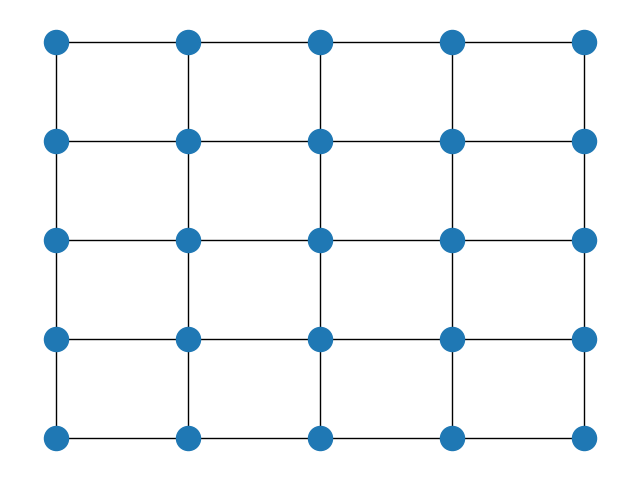Note

This documents the development version of NetworkX. Documentation for the current release can be found here.Out:

(0, 0) (1, 0) (0, 1)
(0, 1) (1, 1) (0, 2)
(0, 2) (1, 2) (0, 3)
(0, 3) (1, 3) (0, 4)
(0, 4) (1, 4)
(1, 0) (2, 0) (1, 1)
(1, 1) (2, 1) (1, 2)
(1, 2) (2, 2) (1, 3)
(1, 3) (2, 3) (1, 4)
(1, 4) (2, 4)
(2, 0) (3, 0) (2, 1)
(2, 1) (3, 1) (2, 2)
(2, 2) (3, 2) (2, 3)
(2, 3) (3, 3) (2, 4)
(2, 4) (3, 4)
(3, 0) (4, 0) (3, 1)
(3, 1) (4, 1) (3, 2)
(3, 2) (4, 2) (3, 3)
(3, 3) (4, 3) (3, 4)
(3, 4) (4, 4)
(4, 0) (4, 1)
(4, 1) (4, 2)
(4, 2) (4, 3)
(4, 3) (4, 4)
(4, 4)


import matplotlib.pyplot as plt
import networkx as nx

G = nx.grid_2d_graph(5, 5)  # 5x5 grid

print(line)
# write edgelist to grid.edgelist
nx.write_edgelist(G, path="grid.edgelist", delimiter=":")